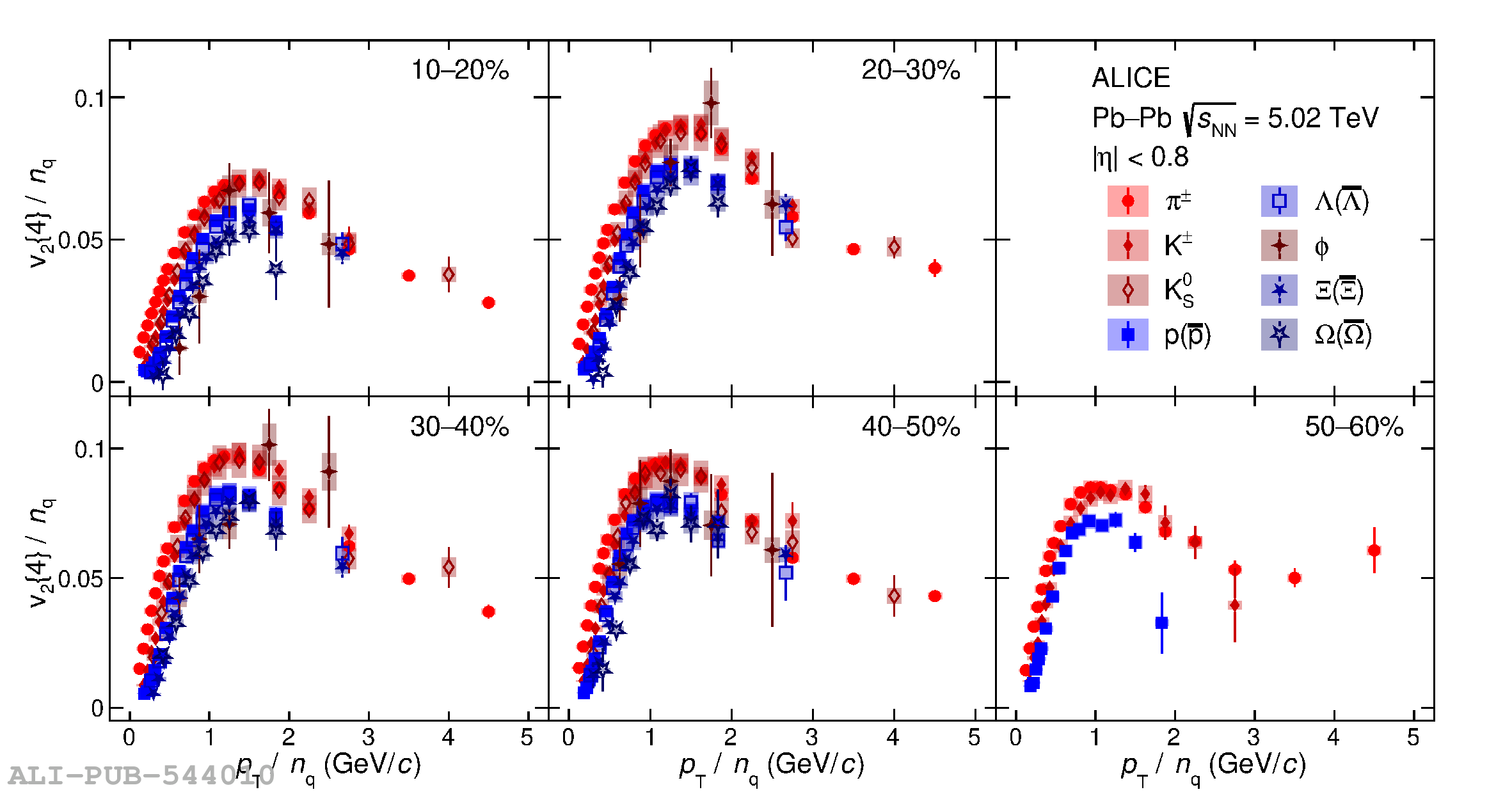# Figure 4

 The dependence of $v_2\{4\}/n_{\rm q}$ on $\pt/n_{\rm q}$, where $n_{\rm q}$ is the number of constituents quarks, for different particle species and centralities in Pb--Pb collisions at $\sqrt{s_{\mathrm{NN}}} = 5.02$ TeV. The vertical error bars and the filled boxes represent statistical and systematic uncertainties, respectively.